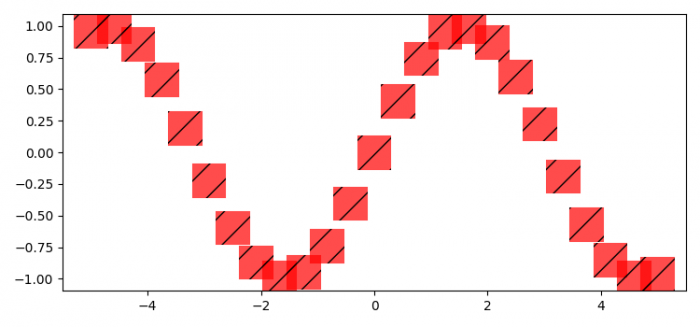# How to change the linewidth of a hatch in Matplotlib?

To change the linewidth of a hatch in matplotlib, we can set the linewidth of the hatch in the params.

## Steps

• Set the figure size and adjust the padding between and around the subplots.
• Create x and y=sin(x) data points using numpy.
• Set the linewidth of the hatch in the plot.
• Plot x and y data points using scatter() method with a square marker having "/" hatches with set linewidth.
• To display the figure, use show() method.

## Example

import numpy as np
from matplotlib import pyplot as plt
plt.rcParams["figure.figsize"] = [7.50, 3.50]
plt.rcParams["figure.autolayout"] = True
x = np.linspace(-5, 5, 25)
y = np.sin(x)
plt.rcParams['hatch.linewidth'] = 1
plt.scatter(x, y, s=700, marker='s', linewidth=0.05, facecolor='red',
hatch='/', alpha=.7)
plt.show()

## Output